Next: Efficient qualitative analysis Up: Analysis of QPNs Previous: Qualitative analysis of

## Quantitative analysis of QPNs

Quantitative analysis of QPNs can be performed by analysis of the embedded Markov chain specified by the QPN's stochastic process. This procedure is analogous to the quantitative analysis of GSPNs . In the following we assume that the QPN is bounded, implying a finite state space. The state space of a QPN is the disjunct union of tangible and vanishing states,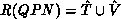where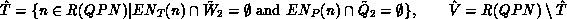Let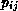denote the probability of changing from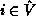to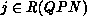,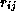be the rate transiting from state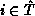toand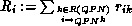.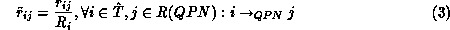specifies the corresponding probability. Define four matrices.

Since the sojourn time in vanishing states is zero by definition, we are only interested in tangible states. Thus it is sufficient to solve the global balance equations of the reduced embedded Markov chain given by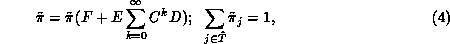providedexists. If (4) has a unique solution the steady state distribution of the QPN's stochastic process is given by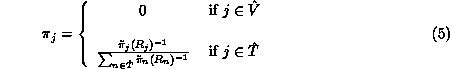Performance measures can be computed from this steady state distribution as usual.

The description above shows that two important preconditions have to be satisfied for a successful quantitative analysis of QPNs:

a)must exist and
b)
Equation (4) must have a unique solution.

Both requirements can be expressed in terms of qualitative properties of the QPN. The existence of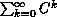corresponds to the notion 'trap'.

Define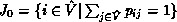and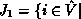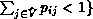, i.e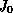and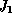contain all vanishing states for which the sum of elements of the corresponding row in matrixequals one or is smaller than one resp. Note that, being in a vanishing state, a tangible state can only be reached via some state of.In QPN terminology this condition is specified by: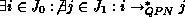.Matrixcharacterizes the changes between vanishing states. Such states are determined by enabling of immediate transitions or scheduling in immediate queueing places according to the QPN description. Thus, if matrixcontains a trap this indicates the possible occurrence of an infinite firing of immediate transitions or in pathological cases an infinite number of actions in an immediate queueing place. Therefore we will refer to this situation calling it a 'timeless trap'. Figure 6 is meant to illustrate a timeless trap caused by never ending firings of immediate transitions. The next theorem proves that in a live QPN the stochastic process can not be "caught" in such timeless traps.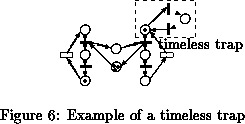implying that matrixhas no trap.
Proof: Assume there is a trap, i.e..
Consider the case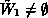. Choose an arbitrary transition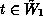and a colour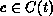. Liveness yields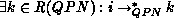and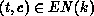and. Thus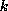is a tangible state which can only be reached fromvia some state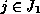.
In the case of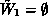choose an arbitrary timed queueing place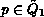,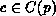and a transition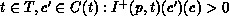. Liveness implies that there exists an infinite firing sequence containing, thus colourtokens are fired infinitely often onto place. From (#>) it follows, that the token number of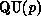increases. Because of our assumption all states reached from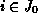are vanishing, thus the internal state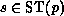ofis only modified by the AC-function, so that the token number ofis not upper bounded, contradicting the boundedness requirement. Note, (#>) implies: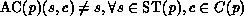.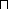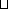=0
So in bounded and live QPNs the existence ofis guaranteed. The second requirement, a unique solution of (4), is satisfied if the state space of the reduced embedded Markov chain contains only one irreducible subset of states, provided the state space is finite. There is only one such subset iff the QPN has at least one home state.

Summarizing all arguments we realize that quantitative analysis of QPNs can be performed by steady state analysis of the reduced embedded Markov chain, if the QPN is bounded, live and has home states. With that, qualitative properties of the QPN are essential preconditions for a successful quantitative analysis.

The next section is dedicated to a more efficient determination of some of these properties by exploiting the PN part of the QPN.Next: Efficient qualitative analysis Up: Analysis of QPNs Previous: Qualitative analysis of

bause
Tue Jan 9 09:36:45 MET 1996